• ## plot颜色设置

千次阅读 2018-07-01 19:04:39
怎么分别设置点和线条的颜色？p.plot(color=''r, secondary_y=True, style='-*', linewidth=2)怎么把点和线设置为两种不同的颜色?
怎么分别设置点和线条的颜色？
p.plot(color=''r, secondary_y=True, style='-*', linewidth=2)怎么把点和线设置为两种不同的颜色?
展开全文python matplotlib
• 官网超棒 1.设置正常中文字体 mpl.rcParams['font.sans-serif'] = ['KaiTi'] mpl.rcParams['font.serif'] = ['KaiTi'] ...2.设置背景和绘图区颜色 plt.rcParams['axes.facecolor'] = 'azure' ...
官网超棒 1.设置正常中文字体
mpl.rcParams['font.sans-serif'] = ['KaiTi']
mpl.rcParams['font.serif'] = ['KaiTi']
mpl.rcParams['axes.unicode_minus'] = False
2.设置背景和绘图区颜色
plt.rcParams['axes.facecolor'] = 'azure'        #设置绘图背景色
plt.rcParams['figure.facecolor'] = 'azure'      #设置背景色
3.设置可变颜色 色卡 在plot/scatter的颜色参数处输入一个列表，其中数值大小代表颜色的相对值，数值越小颜色越深，可将列表导致使较大值输出的颜色较深一点（不能自己指定颜色）。同时，数值相同的点颜色一样。 3-1 设置可变大小，传入列表，值越大点越大
a = [1,2,3,4,5,6,7,8]
b = [1,2,3,4,5,6,7,8]
c = [10,20,30,40,50,60,70,80]
color = c
color.reverse()
plt.scatter(a,b,s = 70,marker = 'o',facecolor='g',c = color)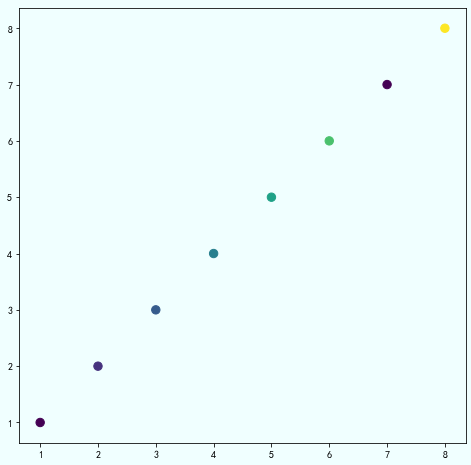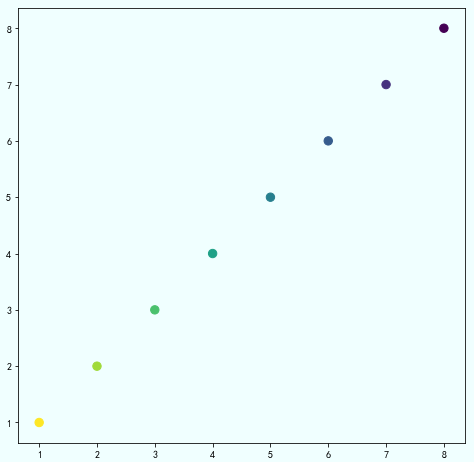4.设置坐标轴、标题、图例
    plt.title(label +': 时间-压力 '+op+'指数平滑曲线', fontsize=18)
plt.xlabel('时间', fontsize=15)
plt.ylabel('压力', fontsize=15)
plt.legend(('原数据', '一次平滑', '二次平滑','三次平滑'), loc='best')
5 保存图片 设置保存路径，像素（dpi）
plt.savefig('test2.png',dpi = 300)
展开全文• 转自：https://blog.csdn.net/jirryzhang/article/details/77374702 MATLAB plot画线的颜色设定 plot中画线的颜色通常是八种： 标记符 颜色 r 红 g 绿 b 蓝 c 蓝绿 m 紫红 y ...
转自：https://blog.csdn.net/jirryzhang/article/details/77374702
MATLAB plot画线的颜色设定 plot中画线的颜色通常是八种： 标记符     颜色 r               红 g              绿 b              蓝 c              蓝绿 m            紫红 y              黄 k              黑 w             白
但是线的颜色是由红绿蓝（RGB）三原色组成的，通过设定三原色的权重可以改变线的颜色，命令如下（x为横坐标，y为纵坐标）：  color的域值为0—1  plot(x,y,'Color',[1 0 0]);代表红色 plot(x,y,'Color',[0 1 0]);代表绿色 plot(x,y,'Color',[0 0 1]);代表蓝色  plot(x,y,'Color',[0.3 0.8 0.9]);则是三种颜色组成的新颜色。 当需要以上常用的八种以外的颜色时，只要设置颜色中RGB的值就可以得到不同的颜色。
比如画一条蓝色的x号线: plot(x,y,'bg') 画图： 线形：－实线 －. 点划线 －－长虚线 ：短虚线 符号 颜色   符号    线形 b       蓝        .         点 c       青          。     圈 g       绿       ×      ×标记 k      黑         －       实线 m     紫红   *         星号 r        红       ：       点线 w     白      －.      点划线 y      黄    －－     虚线 ---------------------  作者：jirryzhang  来源：CSDN  原文：https://blog.csdn.net/jirryzhang/article/details/77374702   
展开全文• matlab开发-plotbar3颜色指示sxyz。三维条形图，将条形高度编码为颜色
• 目的：将matlab二维数组通过plot3形象的三维图表达 成果图如上图所示，黑色的线，红色的线和绿色的线分别代表数据集中的三个标签类别，横轴代表样本总数90个（图中没有截全，只能看到40左右），纵轴代表每个样本的...
目的：将matlab二维数组通过plot3形象的三维图表达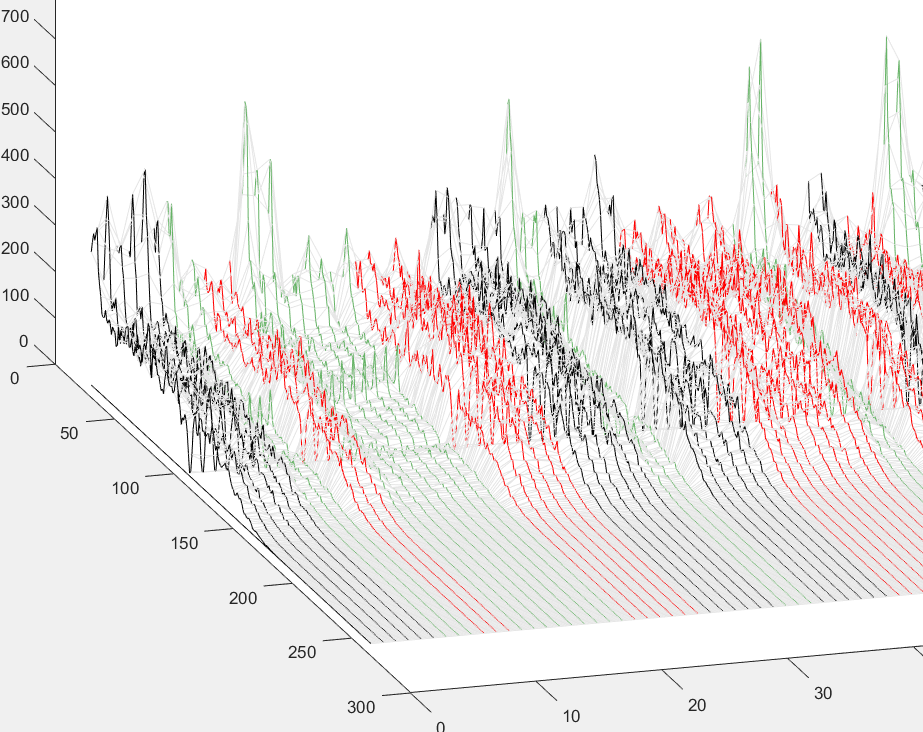成果图如上图所示，黑色的线，红色的线和绿色的线分别代表数据集中的三个标签类别，横轴代表样本总数90个（图中没有截全，只能看到40左右），纵轴代表每个样本的长度256个点。
主要实现函数（plot3）
步骤分为：
1.绘出plot3的图形
2.根据分类标签改变图形的颜色
步骤一：绘出plot3的图形
不熟悉plot3函数的朋友们可以先使用帮助help或doc plot3 了解的matlab自带例程，然而会发现例程中直接使用函数cos()，而没有用一个现成的数组。
因而我们需要自己写个XY网格，在将我们的数组换成对应的Z。
为方便大家理解代码，增加了变量bios=n，用于移除每个样本前n个数值。 数据样本设置：data_all=90 × 256 double
bios=10;     %移除数据样本中前bios个数据，不需要移除数据设置为0就行
[X,Y] = meshgrid(bios:size(data_all,2),1:size(data_all,1));   %建立XY网格
Z = data_all(:,bios:end);
plot3(X,Y,Z,'color',[0.5 0.5 0.5])   %绘制plot3图，设置全部颜色为灰

运行结果如下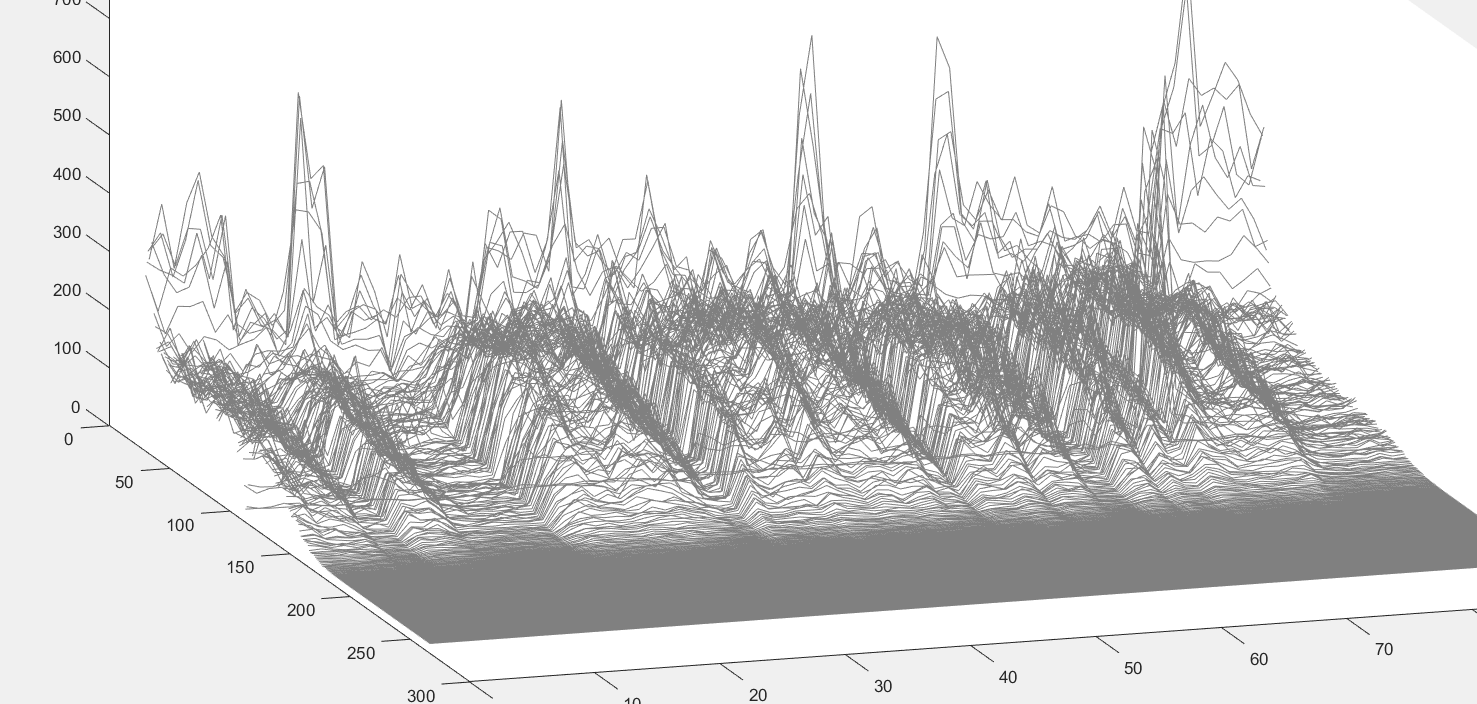步骤二 .根据分类标签改变图形的颜色
这里我通过多次调用plot3实现了改变局部颜色的重绘覆盖，然而这个方法虽然实现了功能，但显然可以看出其效率不高，希望各路大神指点。
接上面的代码继续： 变量说明：label为我90个数据样本的标签集，大小为：90 × 1 double，数据只为1,0或者-1
 for i=1:numTrials%遍历每个样本
hold on
if(label(i) == -1)%%标签如果为-1
Z = data_all(i,bios:end);
plot3(X(i,:),Y(i,:),Z,'k')   %%当前样本颜色重绘为黑色

else if(label(i) == 1 ) %%标签如果为1
Z = data_all(i,bios:end);
plot3(X(i,:),Y(i,:),Z,'r') %%当前样本颜色重绘为红色

else if(label(i) == 0)%%标签如果为0

Z = data_all(i,bios:end);
plot3(X(i,:),Y(i,:),Z,'color',[0.4 0.7 0.4])%%当前样本颜色重绘为浅绿色
end
end
end
end

运行结果如下：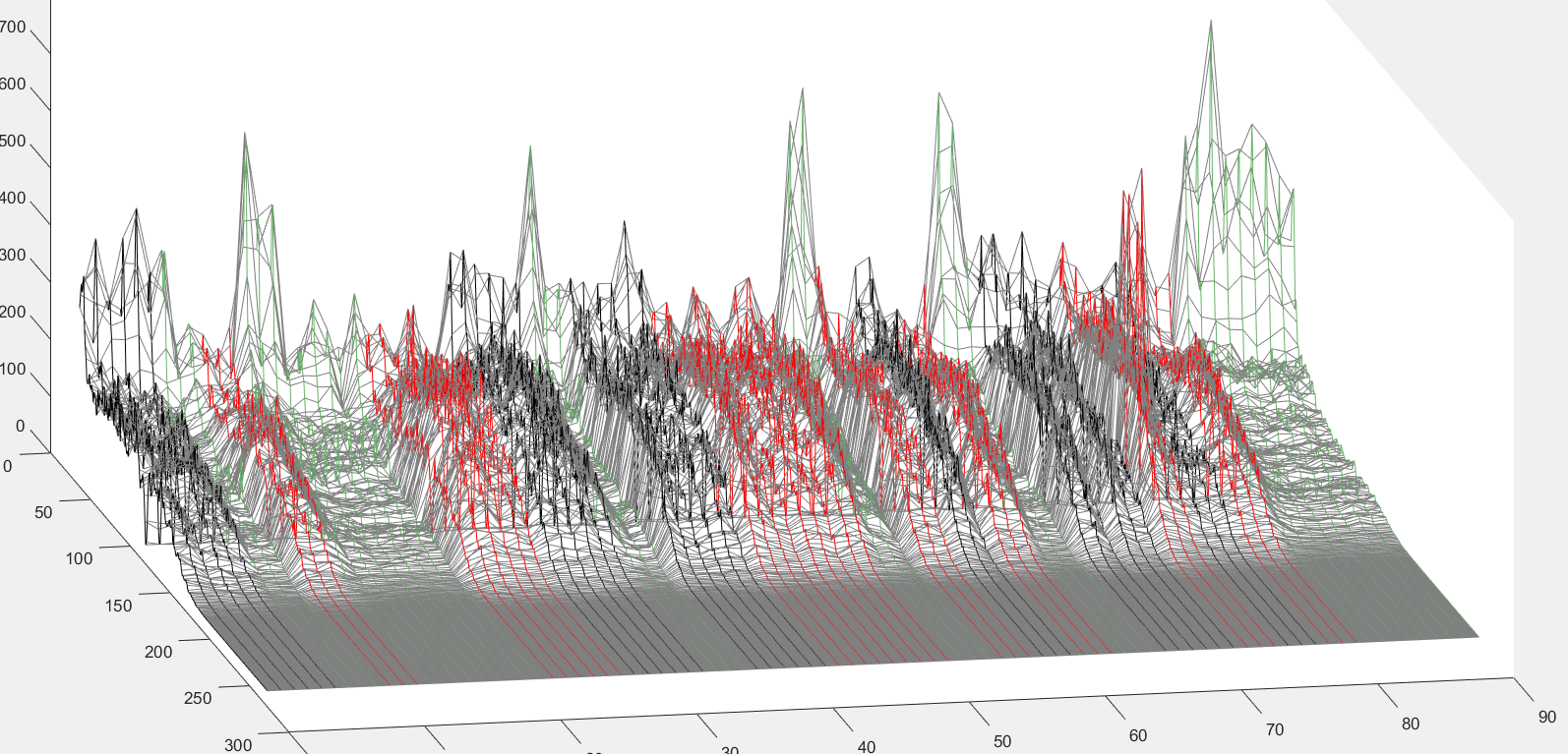不足： 可以看到这个方法只改变了纵向（样本数据）线条的颜色，而横向（样本个数）线条的颜色并未改变。某菜在这里抛砖引玉，希望诸位大佬能够提出更好的解决方法~
展开全文matlab 三维绘图 二维数组
• plot（x,y,'r--')% r为颜色，--为线形
• 这个需求看起来很拗口，不过也是...另外关于颜色设置，由于目前matlab可以自己选择一些人类看起来舒服、区分度较高的颜色，我已经很久不自己指定曲线颜色了。搜了一下，发现并不是我一个人有标题中的需求，例如这里： ...
• matlab plot命令：形如plot（x,y1,'p')；matlab
• 3）Color：颜色 （4）MarkerType：标记点的形状 （5）MarkerSize：标记点的大小 （6）MarkerFaceColor：标记点内部的填充颜色 （7）MarkerEdgeColor：标记点边缘的颜色 1、线形
• help plot中： b blue . point - solid g green o circle : dotted r red x x-markmatlab c
• plot(x,y,'Color',[R G B]);%只要设置颜色中RGB的值就可以得到不同的颜色。Red(红)Green(绿)Blue(蓝)颜色000黑111白100红010绿001蓝110黄101洋红011青蓝0.6670.6671天蓝10.50橘黄0.500深红0.50.50.5灰色...matlab RGB
• clear t=0:pi/20:2*pi; plot(t,sin(t),'--mo','LineWidth',2,'MarkerEdgeColor','k','...%'--mo',这里是设置线型（--）和颜色（m），标记点类型(o，没有变默认为连续），后面一次是线的宽度（'LineWidth',2），...matlab
• MATLAB Plot 如何自定义颜色 Tip: Matlab 作图时最好保存成fig格式，便于以后修改。 写论文是一个漫长的过程，成文后最重要的一个问题就是如何让自己的论文排版和插图更加的好看，让枯燥的论文有一点生动的色彩。...
• 1.转载：... plot(x,y,'Color',[R G B]);%只要设置颜色中RGB的值就可以得到不同的颜色。 2.转载：https://blog.csdn.net/guyuealian/article/details/78845031 将数据x归一化到任...
• Plot是matlab里常用的命令~ 可以通过help plot来查询关于plot绘图的相关命令 [c#]view plaincopy helpplot 推荐大家自己画画图测试一下~ ...而且这些命令是可以叠加使用的。...除了matlab自带的这8种颜色，如果需...matlab
• 3）Color：颜色 （4）MarkerType：标记点的形状 （5）MarkerSize：标记点的大小 （6）MarkerFaceColor：标记点内部的填充颜色 （7）MarkerEdgeColor：标记点边缘的颜色 1、线形MATLAB
• ## 转：plt.plot的颜色

万次阅读 2018-07-17 19:10:29
plt.plot(x, y, linewidth = '1', label = "test", color=' coral ', linestyle=':', marker='|') plt.legend(loc='upper left') plt.show() 结果： 说明：其实上面color=' coral '中，可以换成color=' ...
• ## plot

2018-10-27 23:01:37
2.plot(X,Y,LineSpec) 设置线条样式、标记符号和颜色3.plot(X1,Y1,...,Xn,Yn)对所有线使用相同的轴绘制多个x，Y对。 4.plot(X1,Y1,LineSpec1,...,Xn,Yn,LineSpecn) 设置每一行的线条样式、标记类型和...matlab hopfield
• matlab开发-plot3c。绘制以颜色编码的三维数据。
• 常用顶上 代码： plt.subplots(1, 1) x= range(100) y= [i**2 for i in x] plt.plot(x, y, linewidth = '1', label = "test", ...如下： plt.plot([1,2], lw=4, c=seaborn.xkcd_rgb['baby poop green']) 所有颜色如下：
• import pandas as pd df1 = pd.read_csv('/home/sc/Desktop/2018-5-21/2018-7-18/contract_company_predict_03.csv') # df2 = df1.groupby('shixin_risk_rank_actual').size() df2 = df1.groupby('bankrupt_risk_ran...
• 程序包括二维（Plot），对数（loglog）,分布图（histogram），柱状图（bar），双Y轴图（plotyy），阶梯图（linstairs），栅格（grid），三维（3DPlot）,极坐标图（PolarPlot），等高线图（meshc），以及色彩，线型...matlab
• （matlab)plot画图的颜色线型         y         黄色           ·  ...MATLAB
• figure('color',[1 1 1]) //控制图片背景颜色为白色（这个要写在plot之前） plot(x,y1,'-o',x,y2,'-*') //画图啊，o和*是线型，公用x轴，两个y值，就是两条线 xlabel('x轴') //控制x轴标题...
• PLOTA 与标准 PLOT 函数相同，但它使用其他颜色绘制每个新图（自动“保持”并按照当前轴 ColorOrder 属性指定的顺序循环显示颜色） 例子： 数字; plota(randn(1,100)); plota(randn(1,100)); plota(randn(1,100)); ...matlab
• plot画图时可以设定线条参数。包括：颜色、线型、标记风格。 1）控制颜色 颜色之间的对应关系为 b---blue c---cyan g---green k----black m---magenta r---red w---white y----yellow 有三种表示颜色的方Python
• （matlab)plot画图的颜色线型  y 黄色 · 点线  m 粉红 ○ 圈线  c 亮蓝 × ×线  r 大红 ＋...